## Mihai Prunescu: Symmetries in the Pascal triangle: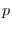-adic valuation, sign-reduction moduloand the last non-zero digit, 431-447

### Abstract:

Consider a prime number. Let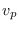be the-adic valuation. Let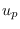be the sign reduction modulodefined as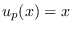if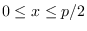and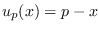if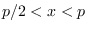. We say that a triangular numeric pattern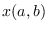with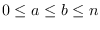has triangular symmetry if it is preserved by the dyhedral group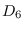. We show the following facts about binomial coefficients:
1.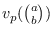build a pattern with triangular symmetry for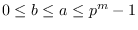.
2.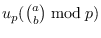build a pattern with triangular symmetry for.
3.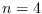is the only composite number such that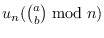has triangular symmetry for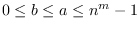. The fact that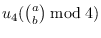has triangular symmetry was previously observed by A. Granville.
4.applied to the last non-zero digit of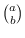represented in the number system with basebuilds a pattern with triangular symmetry for.
Finally, a combined pattern unifies all proven features.

Key Words: Binomial coefficient,-adic valuation, triangular symmetry, Kummer's theorem about carries, Pascal's Triangle modulo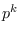, automatic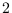-dimensional sequence, Zaphod Beeblebrox.

2010 Mathematics Subject Classification: Primary 11A07; Secondary 05E11, 28A80, 68Q45.